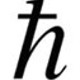# Diminishing Returns for Druids

This will be a quick post, as I’m pressed for time and leave for vacation tomorrow. However, Hinalover provided level 85 and 90 data sets for druids, and I wanted to get the results posted before I leave. The files are available as usual: dr_eqn_druids.m dr_eqn_druids.mat

This time around, things are quite a bit easier. I already know that the agi to dodge conversion factor at level 85 is 243.58281085, and Ghostcrawler recently verified that the level 90 conversion factor is 951.158596. In light of the paladin and monk results, it seems reasonable to guess that this is the conversion factor for all classes (agi->dodge for monks, str->parry for paladins/warriors/DKs).

Without further adieu, here are the fits and plots at 85:

     General model:
lvl85_fit2(x,y) = 3.00+91/243.58+1/(1/C+k/(y+(x-91)/243.58))
Coefficients (with 95% confidence bounds):
C =       150.2  (149.1, 151.4)
k =       1.222  (1.22, 1.223)

lvl85_gof2 =

sse: 0.0033
rsquare: 1.0000
dfe: 32
rmse: 0.0101And for level 90:

     General model:
lvl90_fit(x,y) = 3.00+102/951.158596+1/(1/C+1.222/(y+(x-102)/951.158596))
Coefficients (with 95% confidence bounds):
C =       150.3  (150.1, 150.6)

lvl90_gof =

sse: 2.0846e-004
rsquare: 1.0000
dfe: 28
rmse: 0.0027I actually performed two fits on the level 90 data set, first leaving $k$ as a free parameter, but the fit was just as tight as the level 85 data, making it very clear that $k=1.222$. Fixing that parameter narrowed down the range of $C$ values considerably.

Conclusions:

The diminishing returns equation for druids is of the now-familiar form:

$\LARGE {\rm totalDodge} = {\rm baseDodge} + \frac{\rm baseAgi}{a} + \left (\frac{1}{C}+\frac{k}{{\rm preDodge}+\frac{{\rm Agi}-{\rm baseAgi}}{a}}\right )^{-1}$

with

${\rm baseDodge}=3.00$
${\rm baseAgi}=102$ (presumably race-dependent, not sure which race this was)
$k=1.222 \pm 0.001$ (or less)
$C=150.3 \pm 0.2$
and ${\rm preDodge}$ being the usual pre-DR dodge value due to rating (from the tooltip)

If we really need to narrow the range on $C$, we’d need more data with low agility and high dodge rating to fill in the lower left portion of the surface. Basically, with any fitting operation you want to sample as much of the surface as possible, so getting data points all over the place will increase your accuracy.

This entry was posted in Tanking, Theck's Pounding Headaches, Theorycrafting and tagged , , , , , , , , . Bookmark the permalink.

### 5 Responses to Diminishing Returns for Druids

1.Hinalover says:

race was Worgen

2.tangedyn says:

If it is Worgen, shouldn’t baseAgi be 97, same as trolls as GC mentioned?
Is 102 buffed? I’m quite sure that baseAgi should not include buffs.

•Theck says:

Base stats vary from race to race. I don’t know what base Agi is for Worgen, offhand, but I trust Hina’s ability to read numbers off of the character sheet. 😛

•Hinalover says:

the 102 buffed was actually with Heart of the wild’s 6% Agility buff. I can run tests without HotW talented.

•Theck says:

No need to re-run the tests, as long as you know the untalented baseAgi. It’ll just change the 102 to 96 or 97 (102/1.06=96.22). That will have a slight affect on the coefficients we get from the fit.